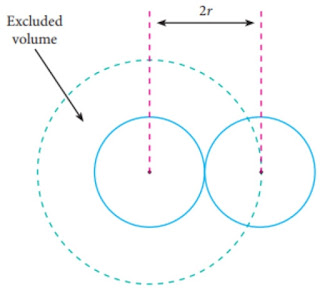# Van der waals Equation of state | Derivation and Correction

Derivation, Correction for the Inter-Molecular Attraction, Correction for the Finite size of the Molecules, Vander Waal's Constant a and b

## ▶Vander Waal's Equation

While deriving the equation of state of ideal gas, it is assumed that the volume occupied by the molecules themselves is negligible compared with the total volume of the gas, and that the molecules exert no appreciable forces on each other. It is evident that both these exemptions cannot be exactly true for actual gases particularly at high pressures. In deriving Vander Waal equation of state the effect of both these factors is taken into account.Excluded Volume

## ▶Correction for the Inter-Molecular Attraction

A molecule in the interior of gas is attached by other molecules in all directions and hence the net cohesive force on it is zero. But when a molecules strikes the wall of the container, it is pulled back by other molecules and has the observed pressure of the gas at the walls of the container is less than the actual process within the body of the gas. This decrease in pressure P proportion to

1. the number of attracting molecules per unit volume,
2. the number of attracted molecules striking per unit area of the wall of the container per second.

Both these factors depend upon the number molecules per density of the gas.

Decrease in pressure

p ∞ ρ2

p = cρ2

where 'c' is a constant and density is given by

ρ= m/V

p=c\frac{m^2}{V^2}

p=\frac a{V^2}

Where (a) is a constant and (V) is the volume of the gas.

Hence real pressure = (P + p)

pressure=\left(P+\frac a{V^2}\right)

Where P is the observed pressure.

Therefore, instead of P, we should write in the equation of state expression

\left(P+\frac a{V^2}\right)

## ▶Correction for the Finite size of the Molecules

As the molecules have finite size they occupy a space. Hence, the actual space for their movement is less than the observed volume (V) of the container. Therefore, the volume V in the equation of state be written as (V - b) where b is a constant for unit mass of a gas.

Thus Vander Waal's equation of a state for a gas is

\left(P+\frac a{V^2}\right)\left(V-b\right)=RT

Where a and b known as Vander Waal's constant and are different for different gases. This is the simplest and most well known equation of state for real gases.

Vander waal's equation of state for n moles is

\left(P+\frac{an^2}{V^2}\right)\left(V-nb\right)=nRT